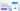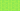Pricing# How to calculate hedge ratio

Written by

Last editedOct 20202 min read

When you're overseeing your investment portfolio, risk management is one of the most crucial elements to consider. That's why hedging – a financial instrument that you can use to mitigate risk – plays a fundamentally important role in managing your exposure. Fair value hedges and cash flow hedges are examples of hedging in practice. But once you've implemented a hedging strategy, how can you work out what percentage of your portfolio is protected from risk? That's where the hedge ratio comes into play. Find out everything you need to know about how to calculate hedge ratio, right here.

## What is a hedge ratio?

Hedge ratio is the comparative value of an open position's hedge to the overall position. A hedge ratio of 1, or 100%, means that the open position has been fully hedged. By contrast, a hedge ratio of 0, or 0%, means that the open position hasn't been hedged in any way. Understanding the hedge ratio can be immensely beneficial for risk management, as it helps you understand the extent to which changes to the value of your asset or liability will be offset by movement in your hedging instrument.

## Calculating the hedge ratio

The hedge ratio formula is as follows:

Hedge Ratio = Hedge Value / Total Position Value

For example, let's say Company A has a portfolio valued at \$5,000,000 that it wishes to hold onto for the next six months. However, Company A would like to ensure that it's protected against currency risk (i.e., foreign exchange movements between AUD to GBP that may spoil their returns). There are lots of ways that you could hedge your exposure to AUD, such as selling \$2,500,000 forward at £1.15/\$.

At the beginning of the hedge, Company A would have a hedge ratio of 0.5 (meaning that 50% of the portfolio is protected from currency risk). Over the course of the six months, however, their portfolio's value will likely increase or decrease, which will change their hedge ratio. So, if the portfolio increases to \$5,100,000 three months in, your hedge ratio will fall to 0.49 (\$2,500,000 / \$5,100,000). At this point, you can choose to adjust the hedge position or do nothing.

## Understanding the optimal hedge ratio formula

Now that you know a little more about how to calculate the hedge ratio, let's explore the optimal hedge ratio (also known as the minimum-variance hedge ratio). Essentially, the optimal hedge ratio is a risk management ratio that helps you work out the percentage of a hedging instrument, or in other words, the percentage of your portfolio that you should hedge. To put it differently, the hedge ratio tells you where you are, and the optimal hedge ratio tells you where you should be. The optimal hedge ratio formula is as follows:

Optimal Hedge Ratio = ρ x (σs / σf)

Where:

• ρ = Correlation coefficient of changes in your future price and spot price

• σs = Standard deviation of changes in spot price (s)

• σf = Standard deviation of changes in futures price (f)

## How are hedge ratios used?

Most commonly, hedge ratios are used as a risk management tool, as it can help you understand the level of risk that you're exposed to with your investment assets. Furthermore, hedge ratios can enable investors and financial analysts to make better-informed investment decisions, as it's an excellent guideline for estimating the performance of an asset.

## We can help

GoCardless helps you automate payment collection, cutting down on the amount of admin your team needs to deal with when chasing invoices. Find out how GoCardless can help you with ad hoc payments or recurring payments.

Over 70,000 businesses use GoCardless to get paid on time. Learn more about how you can improve payment processing at your business today.

Sales

Contact sales

Support

+61 3 8375 9198